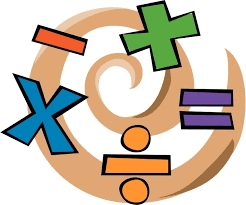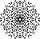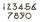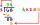# Sixteen

Sixteen minus the quotient of 12 and what number is thirteen?

x =  4

### Step-by-step explanation:Did you find an error or inaccuracy? Feel free to write us. Thank you!Tips to related online calculators
Do you have a linear equation or system of equations and looking for its solution? Or do you have a quadratic equation?
Do you want to perform natural numbers division - find the quotient and remainder?

## Related math problems and questions:

• The quotientThe quotient of g and 55 is the same as 279. What is g?
• Quotient and divisionWhat is the quotient of 2/9 and 1/8?
• RemainderWhat is the remainder of the division of natural numbers 293 and 7?
• What fractionWhat fraction of number 1 to 30 is prime?
• Numbers divisionWith what number should be divided mixed number 2 3/4 to get 11/12?
• Quotient and productIf the quotient of [8/5 divided by 8/10] is added to the product of [8/14 x 7/12 x 3/8], what is the sum?
• DivisionWhich number in division 16 give 12 and the rest 3?
• NumberWhat number do I think? The third is 6, and a half is 2 more than its quarter.
• Find unknown numberWhat is the number between 50 and 55 that is divisible by 2,3,6,9?
• What is 11What is the quotient of Three-fifths and 1 Over 10?
• NumberWhat number should be placed instead of the asterisk in number 702*8 to get a number divisible by 6?
• Result and remainderAfter dividing the unknown number by the number 23, the quotient 11 and the remainder 4 are formed. Find an unknown number.
• DivisibilityDetermine all divisors of number 84.
• Even/odd numbersWhat is the product of 0.97 and the next odd decimal number?
• Quotient 3If the quotient of 8/13 and 2 is subtracted from the product of 1 3/4 and 8/21, what is the difference?
• DivisionDivide by the number 0.2 is the same as multiply by what number?
• Unknown xIf we add to unknown number 21, then divide by 6 and then subtract 51, we get back an unknown number. What is this unknown number?